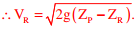# Fluid Mechanics and Thermal Sciences - Online Test

Q1. For steady, fully developed flow inside a straight pipe of diameter D, neglecting gravity effects, the pressure drop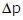over a length L and the wall shear stressare related by
Explaination / Solution: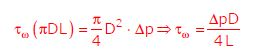Q2. The pressure, dry bulb temperature, and relative humidity of air in a room are1bar, 30ºC and 70% respectively. If the saturated steam pressure at 30ºC is 4.25kPa, the specific humidity of the room air in kg water vapour / kg dry air is
Explaination / Solution: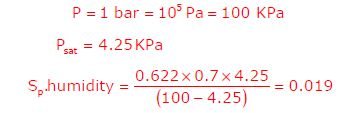Q3. Consider a single degree-of-freedom system with viscous damping excited by a harmonic force. At resonance, the phase angle (in degree) of the displacement with respect to the exciting force is
Explaination / Solution:
No Explaination.

Q4. A mass m1 of 100 kg travelling with a uniform velocity of 5 m/s along a line collides with a stationary mass m2 of 1000 kg. After the collision, both the masses travel together with the same velocity. The coefficient of restitution is
Explaination / Solution: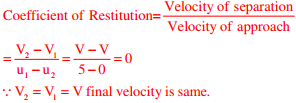Q5. Consider a long cylindrical tube of inner and outer radii, ri and ro, respectively, length, L and thermal conductivity, k. Its inner and outer surfaces are maintained at Ti and To, respectively (Ti> To). Assuming one-dimensional steady state heat conduction in the radial direction, the thermal resistance in the wall of the tube is
Explaination / Solution: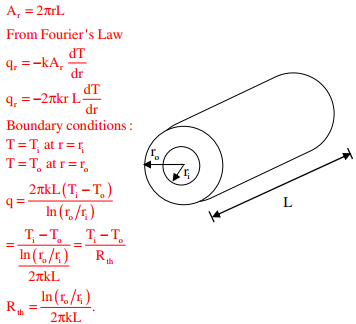Q6. Which one of the following pairs of equations describes an irreversible heat engine?
Explaination / Solution:

For clausius theorem,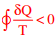(for irreversible Heat engine).

Q7. Consider the turbulent flow of a fluid through a circular pipe of diameter, D. Identify the correct pair of statements.
I. The fluid is well-mixed
II. The fluid is unmixed
III. ReD < 2300
IV. ReD > 2300
Explaination / Solution:

ReD > 2300 means it is a turbulent flow. In turbulent flow, the fluid is well mixed. The fluid is unmixed, for a very-low Reynolds number laminar flow.

Q8. For a gas turbine power plant, identify the correct pair of statements.
P. Smaller in size compared to steam power plant for same power output Starts quickly compared to steam power plant
R. Works on the principle of Rankine cycle
S. Good compatibility with solid fuel
Explaination / Solution:

Steam power plants are bulky due to presence of boiler and condenser. Gas turbines are compact, as compressors and turbines are coupled on a common shaft. In steam power plants, boiler takes lot of time to get started, as compared to Gas Turbines.

Q9. A certain amount of an ideal gas is initially at a pressure P1 and temperature T1. First, it undergoes a constant pressure process 1-2 such that T2 = 3T1/4. Then, it undergoes a constant volume process 2-3 such that T3 = T1/2. The ratio of the final volume to the initial volume of the ideal gas is
Explaination / Solution:

For (1-2)process : Cons tan t pressureprocess {P2 = P1}For (2-3) process : Cons tan t Volume process{V3 = V2}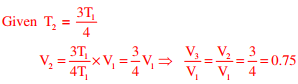Q10. A siphon is used to drain water from a large tank as shown in the figure below. Assume that the level of water is maintained constant. Ignore frictional effect due to viscosity and losses at entry and exit. At the exit of the siphon, the velocity of water is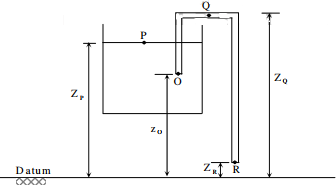Explaination / Solution:

Applying Bernoulli’s equation between the points ‘P’ and ‘R’.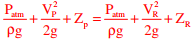Since the level of liquid in tank remains the same, Vp = 0